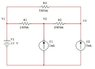# Series and parallel circuit

• Engineering
saulwizard1

## Homework Statement

Hello, can you help with this problem.
For the next circuit, determine the voltages

## Homework Equations

I=V/RT
RT=R1+R2+R3+...+Rn
P=V^2/R

V=V1=V2=V3
I=V/R
GT=1/R1+1/R2+1/R3+...+1/Rn

## The Attempt at a Solution

My background is that I only know a very few and I don´t know how to determine the points and for where to start to solve this circuit.
My idea was try to solve first as a parallel circuit and then as a series circuit.

#### Attachments

•15-7.jpg
10.7 KB · Views: 418